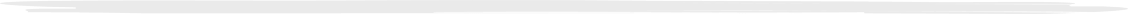## BTU Calculator

Understanding the amount of heat that needs to be generated from a heating appliance is an important factor in determining what size of Heater is needed. Both under-heating and overheating can create an uncomfortable environment. Heaters, when properly sized, should not only take the chill out of an area, but also should not make it uncomfortably warm.

The following is a rough way to figure out a good maximum BTU output for a given space, taking insulation and desired temperature in mind. Please note that there are many factors which affect the maximum area that a Vent Free Heater can service, such as the number of windows and doors, air flow, outside air temperature, total number of exterior walls in the room, etc… Therefore it is generally best to error on the side of a bit larger BTU Vent Free Heater, as it can always be turned off or down to control the heat.

With all this in mind let’s get started.

## What is a BTU?

A BTU is the measure of a heating or cooling appliance’s capacity. An abbreviation for British Thermal Unit, it is the amount of heat needed to heat 1 pound of water by 1° Fahrenheit. As a rough example, the heat generated by burning a wooden kitchen match is 1 BTU. The typical heat gain added to a room by a person at rest is about 230 BTUs per hour.

## What information do I need to have?

First step is to gather some general information that can be plugged into the BTU formula.

1. Determine the Total Cubic Footage of the area that you are trying to heat. The three measurements needed for this are the length, width and height of the space.

Example: Room L 10’ x W 10’ x H 8′ (ceiling height)

2. Determine the level of insulation that the area current has. The three Insulation Levels for our purposes will be:

Tight or Very Good Insulation = 2
Average Insulation = 3
Loose or Poor insulation = 4

The above insulation level (tight, average or loose) will also determine the Insulation Temp Multiplier, as shown below.

Tight or Very Good Insulation = 2.5 Insulation Temp Multiplier
Average Insulation = 3.40 Insulation Temp Multiplier
Loose or Poor insulation = 3.75 Insulation Temp Multiplier

Example: Average insulation = 3
Average insulation temperature multiplier = 3.40

3. Determine the amount by which you would like to raise the temperature in your space.

Example: If it’s currently 50 in your space, and you would like to raise it to 75 then the desired raise in temperature = 25

## And now for the math.

I was told there would be no math! Unfortunately there will be some simple math that must be done in order to come up with a rough estimate of how many BTU’s are required for your space. Grab a calculator, turn it on and plug in your numbers.

## Part two of the formula.

 1. Room Dimension: Width (feet) x Length (feet) x Ceiling Height (feet) = Total Cubic FeetExample: 10 feet by 10 feet with 8 foot ceilings10 x 10 x 8 = 8002. Insulation Level:Tight or Very Good Insulation = 2 Average Insulation = 3 Loose or Poor Insulation = 4Example: I have Average InsulationInsulation Level = 33. Now multiply the above two answers – Total Cubic Feet x Insulation Level =Example: (from above measurements) 800 x 3 = 2400 1. Insulation Temp Multiplier:Tight or Very Good Insulation = 2.50 Average Insulation = 3.40 Loose or Poor insulation = 3.75Example: I have Average InsulationInsulation Temp Multiplier = 3.402. Desired Raise in Temperature:Total raise in Temp / 10 = Desired Temperature.Example: I would like to raise the air temperature 25 degrees.25 / 10 = 2.53. Now multiply the above two answers – Insulation Temp Multiplier x Desired Temp =Example: (from above answers) 3.40 x 2.5 = 8.50